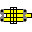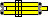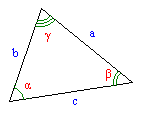#Trigonometry

Typical moderately sophisticated sliderule is usually equipped with 2-3 trigonometric scales. These scales allow to calculate basic trigonometric functions -- sin(x) and tg(x). More complex rules may have up to 10 trigonometric scales that may cover wider variable range for sin/tg, give better precision, introduce another trigonometric functions etc.

Sometimes usage of these scales to solve trigonometric problems is straightforward -- direct application of textbook formulas when slide rule serves just as a multiplication aid. But sometimes there are amazing shortcuts specific to slide rule. This page presents some of the latter.

## Trigonometric scales

Angular units are abundant. Among widely used are: degrees (360 degrees per circle), grads (400 grads per circle), radians (2π radians per circle) and military mils (chosen to be close to milliradian; depdends on country). Here is definition of mil from "http://en.wikipedia.org/wiki/Angular_mil":
The three definitions of the mil

There are 2000×π milliradians in a circle. So a milliradian is just over 1/6283 circle. Each of the definitions of mil are similar to that value but are easier to divide into many parts.

• 1/6400 circle in NATO countries.
• 1/6000 circle in the Soviet Union
• 1/6300 circle in Sweden. The Swedish term for this is streck literally "line". Sweden has not been part of NATO or the Warsaw Pact
By the way, when angle is measured in degrees, fractions of degrees can be expressed as minutes and seconds, or as regular decimal fractions.

As a result of such proliferation trig scales may be marked according to all 8 conventions. I haven't seen Swedish mils on a slide rule yet, but have no doubt such slide rules exist.

Another possibility for variation is scale placement. For example, if slide rule has only 2 trigonometric scale (S and T) there are 2 major possibilities: 1) both of them are located on stock and 2) both of them are located on slide. Operations for these 2 cases may differ. So, to distinguish these cases scales will be denoted TD and SD if they are located on the stock (sines/tangents may be read from D scale) and TC and SC if they are located on the slide (sines/tangents may be read from C scale)

Finally, while most of the time trig scales relate to C/D scales, sometimes sine scale relates to A/B.

You need to keep all this in mind when trying to apply algorithms described in this chapter with your slide rule. Emulator provides scales marked in degrees and decimal fractions of a degree, thus all examples have angular quantities expressed in this form. All algorithms so far rely on S and T scales being related to C and D (rather than A and B).

## Basic trigonometric operations

Ronald Manley has written very good article http://www.sliderules.clara.net/a-to-z/trig-02.htm about solving basic trigonometric problems with different layouts of trig scales. He shows how to calculate
• a * sin B
• a / sin B
• a * sin A / sin B
with
• trig scales on stock;
• trig scales on front of slide;
• trig scales on back of slide with marker on back of rule;
• the differential trig scales on Thornton/PIC rules.
Here are his algorithms written in short notation:
r=a·sinβ r=a/sinβ r=a·sinα/sinβ trig scales on stock1C->βS; aC=>rD aC->βS; 1D=>rC aC->βS; αS=>rC1C->aD; βS=>rD βS->aD; 1C=>rD βS->aD; αS=>rD(flip); βS->1]Y; (flip); aD=>rC βS->1]Y; aC=>rD βS->1]Y; |->aC; αS->1]Y; |=>rCβSd->βD; aC=>rD βSd->βD; aD=>rC βC->αD; |->βSd; αSd->|; aC=>rD a=5, β=36.9°, r=3 a=3, β=36.9°, r=5 a=3, α=30°, β=45°, r=2.12

## Techniques of solving a Triangle

Now lets use above techniques for the arguably most important trigonometry applications -- solving a triangle. To solve a triangle means to determine angles and sides of a given triangle when some of the sides and or angles are given.

### Oblique triangle by side and 2 anglesAmong all triangle solutions the case of oblique triangle with a given side and 2 angles is the easiest. This is because of sine theorem:
a/sinα = b/sinβ = c/sinγ
This immediately leads to the algorithm (assuming S scale is on slide and a, α and β are given): αS->aD; γ:=180-(α+β); βS=>D(b); γS=>D(c)

### Pythagorean theorem (hypothenuse)

For given right triangle with sides a and b its hypothenuse c may be found using formula c2=a2+b2. See
addition page for a direct method of calculation. Here I present "indirect" methods that use trig scales and might be easier to perform on a slide rule.

#### European slide rules: CI,D,TD,SD

From sinα=a/c and tgα=a/b follows c=a/sin(arctg(a/b)).

John Mosand described corresponding slide rule algorithm on 27-Aug-2000 in slide rule mailing list in the following words (assuming that both S and T scales are located on stock):

1. Place C index over D=a.
2. Move the hairline to CI=b.
3. Transfer angle now read on the T scale, to the S scale.
4. Read the result 'c' on CI.
In short notation it may be written as:
or even shorter:(Try this calculation. Use a=3, b=4 then c should be 5)

#### Rules with trig scales on a slide: DI,C,TC,SC

If your rule has T and S scales on the slide but also equipped with DI scale then you may use the same algorithm just "swapping" (in your mind) stock and slide:

aC->1DI;bDI=>T(x);xS=>DI(c)Try with example a=3, b=4 (c=5).

#### DI,TC,SC

Mike Markowski reported the following method which does not use C scale:
1. move index on scale S to a on scale DI
2. move cursor to b on scale DI
3. read x from scale T under cursor
4. move cursor to x on scale S
5. read c from scale DI under cursor

This is equivalent to the previous algorithm because "move slide index to a on DI" puts slide in is exacltly the same position as "move a on scale C to stock index".

#### Common: C,D,TC,SC

The problem with method above is that most common layout (so called "polyphase") has T and S scales on the slide and does not have DI scale.

The best solution for such layout I've come up with requires 2 slide movements: 1C->bD;|->aD;|=>T(x);xS->|;1C=>D(c).

If you know 1 movement solution, please tell it to me.

#### Approximate method (no scales at all)

If a>b then c≈a+b/2. Even better formula is c≈a+b/3 -- at the expence of more difficult to perform mentally operation division by three.

## Miscellaneous problems

I never expected that trig scales can play significant role in solving quadratic equation when Chris Redding sent me the following method.
... I have found a method of solving quadratic equations in the Mathema Manual. It is called the "Goniometric Solution of Quadratics." For real a,b>0, if x2+2ax-b2=0, define μ by tan(2μ) = b/a, then the roots are x1 = b tan(μ) and x2 = - b cot(μ).

... Also, for x2+2ax+b2=0 and b < a, define μ by sin(2μ) = b/a, then the roots are x1 = - b tan μ and x2 = - b cot μ. For the last quadratic, there are no real solutions if b > a. This approach is perfect for the slide rule. But there is a little difference in application depending on where the tangent scale is and how many T scales are present.Suppose we have a FC2/83N with T1 and T2 on the stock. For some x, we have x2 + 234x - 5670 = 0. Then a = 234/2 = 117 and b = sqrt(5670) = 75.2.

1. Place C1.17 over D7.52.
2. Move hairline to C1, read angle T132.7°, 32.7°/2 is 16.4°.
3. Move hairline to T116.4° and place C1 under the hairline.
4. Move the hairline to CF7.52, read DF2.21.
5. Move the hairline to D7.52, read C2.56.
Since 234 > 0 and -5670 < 0, x1 > 0 and x2 < 0. By estimation we find the decimal points and x1 = 22.1 and x2 = -256.

One important point, before we attack ax2 + bx + c = 0, it is prudent to find b2 and 4ac and compare. We don't want to pick up our slide rule and search for roots that aren't there.

### Cubic equation

The following formula follows Viete's reasoning, but is slightly adapted for calculation with a slide rule.

Let's solve equation x3 - p*x + q = 0.

Substitution x=k*sin(t) gives

k3*sin3(t) - p*k*sin(t) + q = 0

Let k=2*3-1/2*p1/2:

8*3-3/2*p3/2*sin3(t) - 2*3-1/2*p3/2 * sin(t) +q = 0

Multiply by 33/2*2-1*p-3/2:

4*sin3(t) - 3*sin(t) + (27/4)1/2*q*p-3/2 = 0

Using formula sin(3*t)=3*sin(t) - 4*sin3(t):

sin(3*t) = (27/4)1/2*q*p-3/2 (*)
t = 1/3 * arcsin((27/4)1/2*q*p-3/2) (**)
and, finally:
x = k*sin(t) = 2*3-1/2*p1/2*sin(1/3 * arcsin((27/4)1/2*q*p-3/2))

(Generally speaking (*) has 3 solutions with (**) being only one of them:

t = 1/3 * arcsin((27/4)1/2*q*p-3/2) + 2*k*π/3, k=0..2
Other 2 solutions involve addition which is not that easy on a slide rule.)

[ Index page | Emulator | Notation | Deframe | Feedback ]